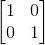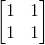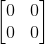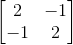Precalculus : Solve a System of Equations Using the Multiplicative Inverse

Example Questions

Example Question #71 : Matrices And Vectors

What is the inverse of the identiy matrix?The identity matrixThe identity matrix